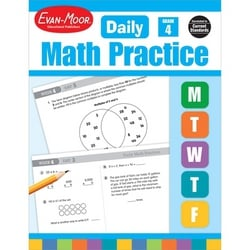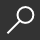{ "item_title" : "Daily Math Practice, Grade 4 Teacher Edition", "item_author" : [" Evan-Moor Corporation "], "item_description" : " Your grade 4 students will build mastery and retention by applying these lessons for as little as 15 minutes a day. Practice computation, word problems, and dozens of other important math skills are covered, all in a range of difficulty appropriate for grade 4. There are 36 weeks worth of lessons, each divided as follows. Practice five items a day Monday through Thursday: two computation problems, two items that practice a variety of math skills, one word problem. Friday's lesson contains a single problem that is more extensive and may require multiple steps. These problems emphasize reasoning and communication in mathematics. Daily Math Practice, Grade 4 includes practice lessons on the following topics: Computation, multi-digit, addition with and without regrouping, multi-digit subtraction with and without regrouping, multi-digit multiplication, division with single-digit divisor, addition and subtraction of fractions. Numbers, base ten system, word/standard forms, place value, rounding, odd/even numbers, ordinals, estimation, properties/ relationships, factors, multiples, equalities/ inequalities, decimals, fractions, integers, Patterns/ Algebra, figural patterns, numerical patterns, expressions, function tables, ratios, Geometry/ Spatial, 2-dimentional shapes, 3-dimentional shapes, symmetry, congruency, spatial, angles, Measurement, weight, capacity, time temperature, length, perimeter, area, volume, money, calendar, Data/Probability, coordinate graphing,, number lines, constructing graphs, interpreting graphs, range, mode, median, probability, permutations/combinations. The page for each day features a place for the student to enter his or her name. All pages are reproducible. Just one example of an exercise found in this book is: What is the median (or middle value) of this data? 44, 48, 52, 54, 58, 63, 65. There is an answer key for each week and a scope and sequence chart. The scope and sequence charts detail the specific skills to be practiced and show when they will be presented. The skills included are those found in math texts at each level. Lessons are illustrated with basic line art of things familiar to any fourth grade student. All 112 reproducible pages perforated for easy removal.", "item_img_path" : "https://covers2.booksamillion.com/covers/bam/1/55/799/744/1557997446_b.jpg", "price_data" : { "retail_price" : "22.99", "online_price" : "22.99", "our_price" : "22.99", "club_price" : "22.99", "savings_pct" : "0", "savings_amt" : "0.00", "club_savings_pct" : "0", "club_savings_amt" : "0.00", "discount_pct" : "10", "store_price" : "" } }Daily Math Practice, Grade 4 Teacher Edition
local_shippingFor DeliveryIn Stock.
FREE Shipping for Club Members help
storeBuy Online Pickup At Storeshow all formats

### Overview

Your grade 4 students will build mastery and retention by applying these lessons for as little as 15 minutes a day. Practice computation, word problems, and dozens of other important math skills are covered, all in a range of difficulty appropriate for grade 4. There are 36 weeks worth of lessons, each divided as follows. Practice five items a day Monday through Thursday: two computation problems, two items that practice a variety of math skills, one word problem. Friday's lesson contains a single problem that is more extensive and may require multiple steps. These problems emphasize reasoning and communication in mathematics. Daily Math Practice, Grade 4 includes practice lessons on the following topics: Computation, multi-digit, addition with and without regrouping, multi-digit subtraction with and without regrouping, multi-digit multiplication, division with single-digit divisor, addition and subtraction of fractions. Numbers, base ten system, word/standard forms, place value, rounding, odd/even numbers, ordinals, estimation, properties/ relationships, factors, multiples, equalities/ inequalities, decimals, fractions, integers, Patterns/ Algebra, figural patterns, numerical patterns, expressions, function tables, ratios, Geometry/ Spatial, 2-dimentional shapes, 3-dimentional shapes, symmetry, congruency, spatial, angles, Measurement, weight, capacity, time temperature, length, perimeter, area, volume, money, calendar, Data/Probability, coordinate graphing,, number lines, constructing graphs, interpreting graphs, range, mode, median, probability, permutations/combinations. The page for each day features a place for the student to enter his or her name. All pages are reproducible. Just one example of an exercise found in this book is: "What is the median (or middle value) of this data? 44, 48, 52, 54, 58, 63, 65." There is an answer key for each week and a scope and sequence chart. The scope and sequence charts detail the specific skills to be practiced and show when they will be presented. The skills included are those found in math texts at each level. Lessons are illustrated with basic line art of things familiar to any fourth grade student. All 112 reproducible pages perforated for easy removal.

• ISBN-13: 9781557997449
• ISBN-10: 1557997446
• Publisher: Evan-Moor Educational Publishers
• Publish Date: January 2014
• Dimensions: 10.86 x 8.54 x 0.36 inches
• Shipping Weight: 0.82 pounds
• Page Count: 128
• Reading Level: Ages 9-10